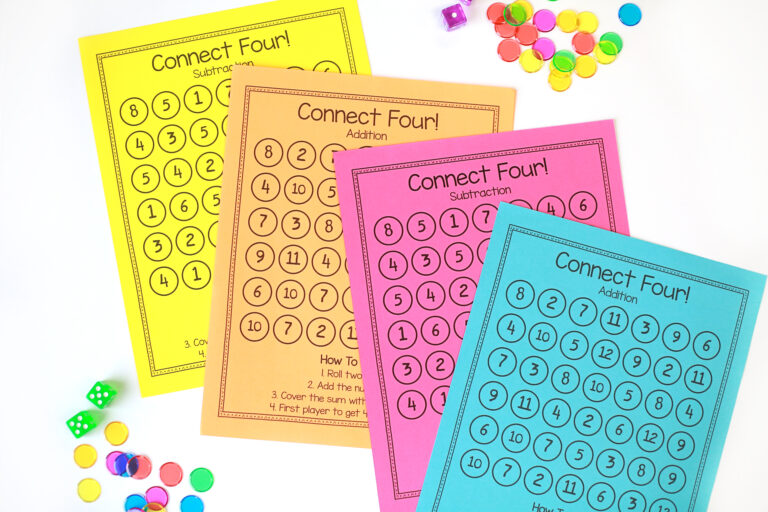Search
Search

# Mental Math Activities & Games for 1st Grade

Mental math is the process of doing mathematical calculations in your head. Students develop this skill with practice. Mental math is a useful tool for students to have. Of course, it can help your learners save time on paper and calculator work, and it can also be used to check their work for mistakes.

Even with beginning math activities for 1st grade, mental math is so important to develop number sense and become more confident with numbers. Finally, these mental math activities and games are designed to give K-2 students practice with addition and subtraction.

By doing these activities regularly, students will become more proficient at mental math. Here are some mental math activities for primary grades to try with your students.

## Mental Math and Number Sense

First, mental math helps students develop number sense, the ability to sense numbers, and have relationships with them. Mental math is also a way to check what students have learned.

Students use mental math to practice and check their work. This helps them become more confident in their understanding of numbers and operations.

## Ten-Frame Mental Math

Ten-frame mental math helps students visualize numbers. It uses ten frames, or dots, to represent numbers from 0-9. Practice addition and subtraction with ten-frames. In these activities, students use the ten-frames to add and subtract within 10. This is a fantastic activity for visual learners and helps students to see the relationship between numbers.

I love using this magnetic ten frame – it’s a wonderful visual and the magnets make it so easy to manage!## Number Line Mental Math

Number lines are another way to practice mental math. First, students can create their own number line using index cards or paper. Of course, you can always add it to your students’ nametags so they have easy access. I recently discovered these number-line whiteboards and they are absolutely perfect for small groups you may pull.

They can use the number line to easily practice addition and subtraction within 10. Try a number line shaped like a path or road. Students start at one end of the number line and add or subtract the numbers they land on until they reach the other end. This way to practice mental math is easily differentiated.

Related Reading: Math Board Games for Kids

## Mental Math Warm Up

Before beginning a lesson or starting a new topic, write a few mental math problems on the board for students to solve. As a class, discuss the different strategies that students used to solve the problems and have students explain their reasoning.

After completing a mental math activity or speed drill, have students share their results with a classmate and explain how they arrived at their answer.

Before I begin any math lesson, we pull a stick from our Math in a Minute bucket. These simple problems are just what you need to warm up those math brains!

Each month is filled with 24 themed problems that spiral through the 1st-grade math standards. They can definitely work for Kindergarten and 2nd Grade, too!

Want to try a free set? Enter your info below!

## Number Train

Another mental math game for helping students learn their number facts is Number Train. Write the numbers 1-9 on the board. First, choose a starting number and then have students take turns calling out the next number in the sequence that is 9 more or less than the previous number.

For example, if the starting number is 5, the next number could be either -4 or 14. The goal is to see how long the “train” can go without derailing. This game can also be played with other number ranges, such as 1-5 or 1-20.

## Number Pattern Puzzles

Number pattern puzzles are another mental math activity. First, cut out number cards and have students arrange them in order from 1-9. Next, have students look for patterns in the numbers and explain the patterns that they see. For example, the numbers go up by 2 and then down by 1. Students can also find the odd and even numbers, as well as the numbers that are multiples of 3.

## What’s My Rule?

This is a great game for practicing number patterns. First, cut out number cards and mix them up. Have students draw a card and place it in the correct spot on the game board. The goal is to figure out the rule for ordering the numbers. For example, the numbers might go up by 2 and then down by 1.

Finally, dominoes are awesome manipulatives for practicing mental math! Have students play this game with a partner. First, each player starts with 5 dominoes.

Next, place the dominoes face down and mix them up. Then take turns flipping over 2 dominoes. Finally, add the numbers on the dominoes and say the answer out loud. The first player to get rid of all of their dominoes wins the game.

If your students can’t get enough domino fun, extend that learning with these Mystery Math Dominoes.

Students need to use dominoes to solve math equations. These dominoes are a great activity to leave in bins for early finishers.

## Recap of Mental Math Games & Activities for 1st Graders

Overall, mental math activities are a great way to help primary students develop their arithmetic skills.

By incorporating these activities into your lesson plans, you can give your students a head start on success in math!

What games do your students like for mental math? Tell me in the comments!

This site uses Akismet to reduce spam. Learn how your comment data is processed.